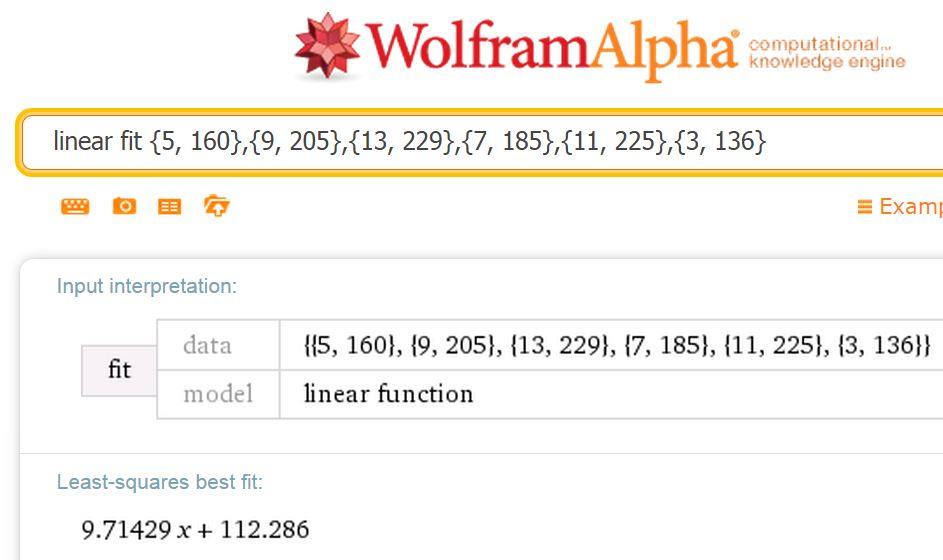# Tidying Up!

A break is always a good time for tidying up and reorganising things!

Continuing the Use of Technology series of pages, the PhET Simulations, Standards Unit Software and Wolfram Alpha pages have been added to the series.

Added to the GeoGebra series is a new page, GeoGebra Resources-Edexcel so all the resources for GCSE and A level Maths and Further Maths are available in one place.

A recent addition to the top menu includes Updates where updates to popular posts are noted. A further new page added today is Popular Posts and Links, just a small number of currently popular posts and/or files. I see that the file of legacy coursework tasks from Edexcel has proved very popular this year as has The Workers of Zen.

# Integration – Desmos & WolframAlpha

With my year 12 students (UK age 16-17) we have been looking at definite Integration. Desmos and WolframAlpha are both excellent for checking work and by using the technology we have a very clear visual representation adding to our understanding.

One of the homework questions for my students involves finding the total shaded area bounded by f(x) = x4−3x3−4x2+12x, the x-axis, the line x=−1 and the line x=3.

We could use WolframAlpha for a quick check. I like the visual representation showing students clearly that they are dealing with areas above and below the x-axis.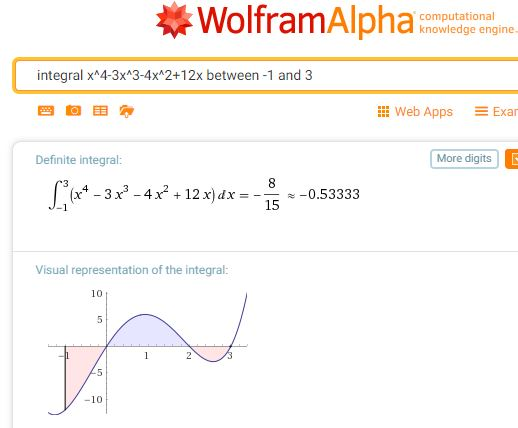Scrolling down the page we see that this query also returns the indefinite integral.For the total shaded area, students could change the limits of the query to evaluate each section.

Or we could turn to the excellent Desmos where we can very simply change the limits.

If you are unfamiliar with Integration with Desmos, turn to Learn Desmos: Integrals.
Note you can explore the graph shown in the video.

A version of this post for students which also includes links to some useful notes and examples can be found on Mathematics for Students.# Low Stakes Testing in the Mathematics Classroom

Please note – a completely new version of this presentation with many more resources and additional ideas will be presented at the BCME Conference, April 2018. Following my sessions, I will update all relevant pages

Low Stakes Testing in the Mathematics Classroom (PowerPoint File, takes a few moments to download)

Or use this shorter version to see the slides on the student survey on low stakes tests.
Mini Tests Colleen Young for PowerPoint

Slides from the BERA Conference, Learning from the classroom – Practitioner research in mathematics education – July 2016

There are many hyperlinks provided in the presentation, for ease of access these are also provided below:

# Solving Linear Equations

A collection of resources to use to demonstrate and practise solving equations.

Student Exercises
I find Owen Elton’s worksheet, Balancing Equations on TES Resources very useful when introducing equations, the diagrams emphasise that we must do the same to both sides.
(See Diagrams in Mathematics, for more on the use of diagrams to help understanding).

As an alternative to the balance approach, consider this doing / undoing approach, described here by Don Steward, this is an approach I use for finding inverse functions. The exercises Don refers to are here.

One of the Standards Unit resources, A2 Creating and solving equations (in Mostly Algebra) uses this approach, students create an equation and  then undo it; this is a great exercise for demonstrating notation. Another resource with this approach comes from the Mathematics Assessment Project (the design and development was led by the MARS Shell Center team at the University of Nottingham) Building and Solving Linear Equations lesson

For a superb collection of ideas and student exercises for solving linear equations see all Don Steward’s posts tagged linear equations. Many of these outstanding resources use a very visual approach with very clear diagrams to help students’ understanding.

There are numerous questions on linear equations on Diagnostic Questions.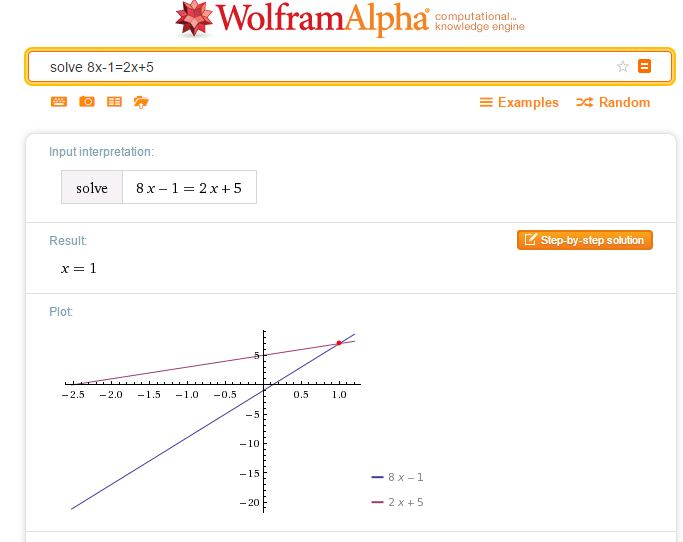A simple way to check a solution to an equation of any type is to simply enter your equation as a WolframAlpha query. Note that WolframAlpha includes a graphical illustration; it is so important for students to understand how equations may be solved graphically; I always illustrate graphical solutions when we are working with Algebra to help students make these links. Desmos of course, is ideal here.Centre for Innovation in Mathematics Teaching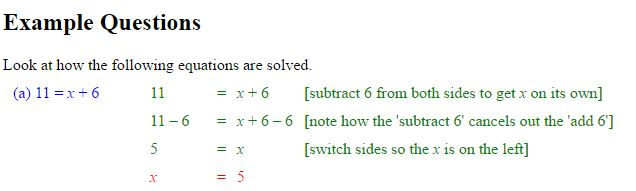CIMT
have tutorials on equations: Linear Equations 1, Linear Equations 2, and Linear Equations with Brackets.

The following three resources work well for demonstrating the balance method of solving linear equations.

Duncan Keith’s Linear Equation Calculator is available on STEM Learning.Choose the type of equation you require then the sequence of operations required to solve the equation.
Select Do it after each operation, for example -32 Do it were the keys selected to start the above problem.

The slideshow below shows how to use the calculator to solve equations where the unknown is on both sides.

Mathisfun

Mathisfun has this very clear and easy to use interactive illustrating the solution of linear equations.

# Regression

Since I will be studying the Bivariate Data section on one of our Year 13 (UK age 17-18) Statistics modules soon I was rather pleased to see that it is now really easy to use Desmos for Regression. The video shows how simple it it to use.

I tried this with some data from an A level question then also added another expression and table to see the values predicted by the model.It is also simple to use Excel – just add the data, insert a chart – choose Scatter, then add a trend line.Regression using Excel

GeoGebra provides us with another option.
Try this ready to use worksheet – find the line of best fit.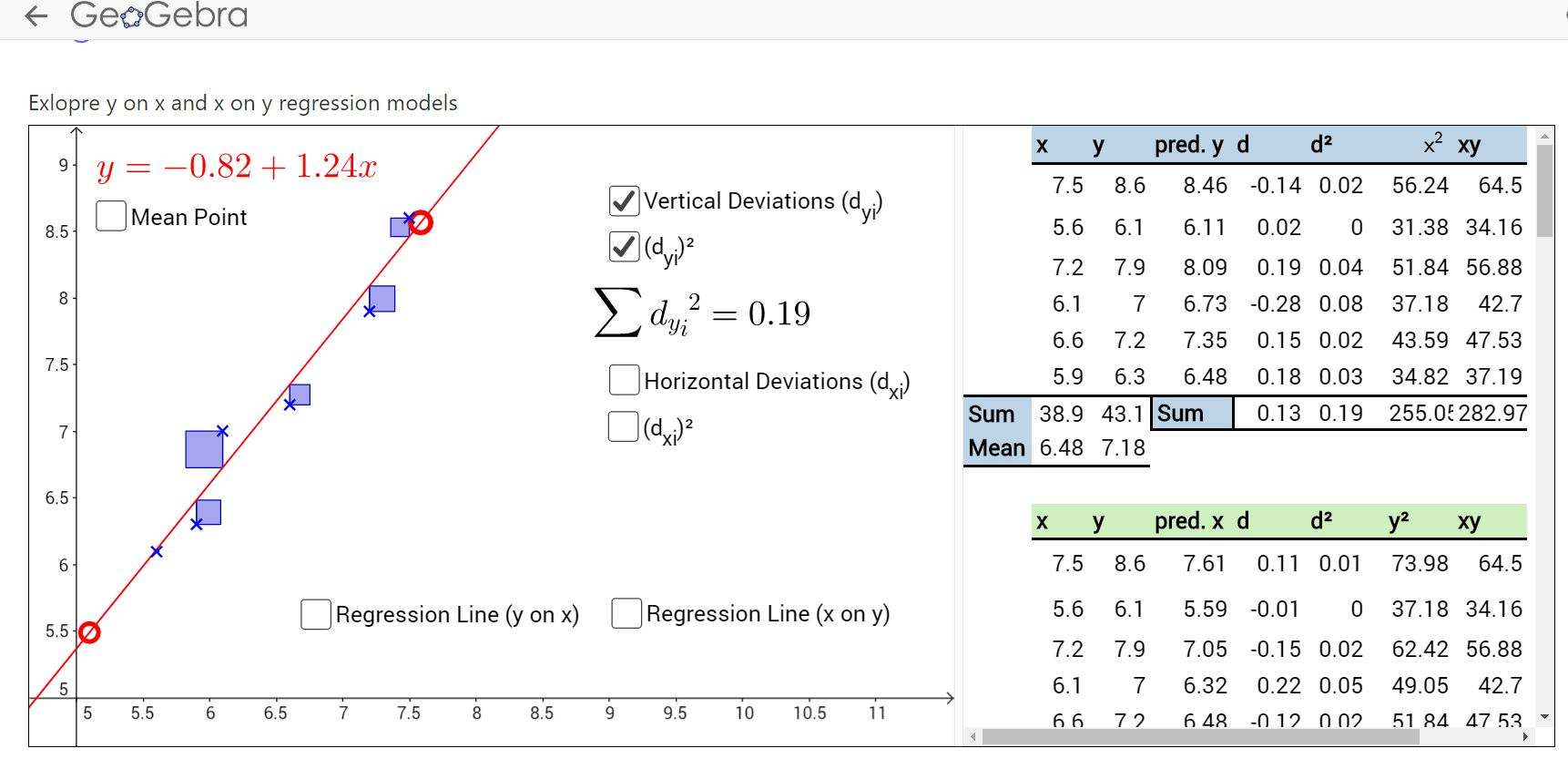From the GeoGebra manual – FitPoly command

For another easy to use tool, we could use the PhET simulation.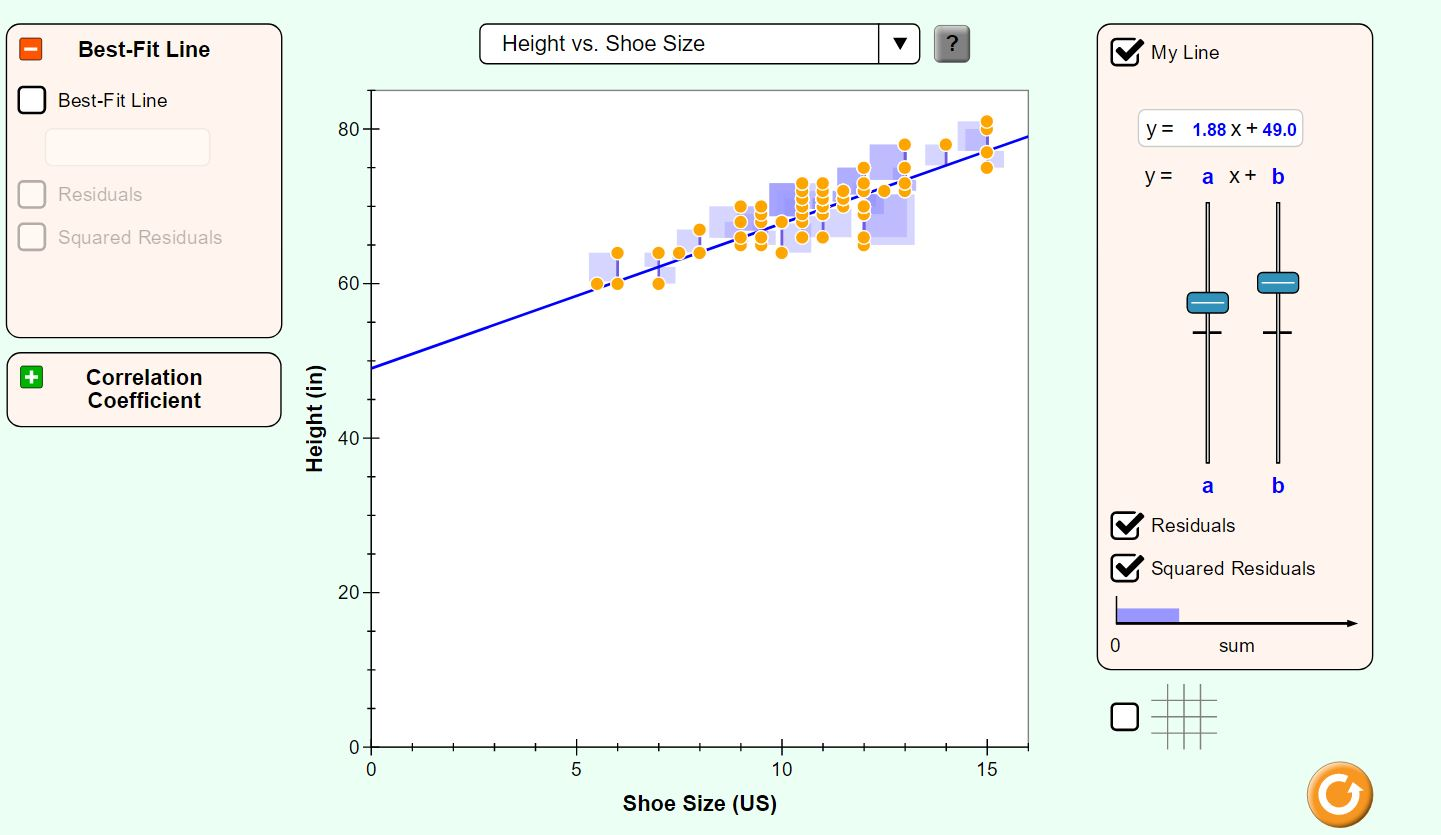…or we could just pop the points into WolframAlpha and have it do all the work to check our calculations!